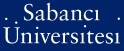# The Camassa-Holm equation as the long-wave limit of the improved Boussinsq equation and of a class of nonlocal wave equations

Erbay, Hüsnü Ata and Erbay, Saadet and Erkip, Albert (2015) The Camassa-Holm equation as the long-wave limit of the improved Boussinsq equation and of a class of nonlocal wave equations. (Accepted/In Press)There is a more recent version of this item available.Preview
PDF (This is a RoMEO yellow journal -- author can archive pre-print (ie pre-refereeing)) - Requires a PDF viewer such as GSview, Xpdf or Adobe Acrobat Reader
231Kb

## Abstract

In the present study we prove rigorously that in the long-wave limit, the unidirectional solutions of a class of nonlocal wave equations to which the improved Boussinesq equation belongs are well approximated by the solutions of the Camassa-Holm equation over a long time scale. This general class of nonlocal wave equations model bidirectional wave propagation in a nonlocally and nonlinearly elastic medium whose constitutive equation is given by a convolution integral. To justify the Camassa-Holm approximation we show that approximation errors remain small over a long time interval. To be more precise, we obtain error estimates in terms of two independent, small, positive parameters ϵ and δ measuring the effect of nonlinearity and dispersion, respectively. We further show that similar conclusions are also valid for the lower order approximations: the Benjamin-Bona-Mahony approximation and the Korteweg-de Vries approximation

Item Type: Article Q Science > QA Mathematics > QA299.6-433 Analysis 29130 Albert Erkip 25 Jan 2016 10:51 23 Aug 2019 15:57

### Available Versions of this Item

Repository Staff Only: item control page